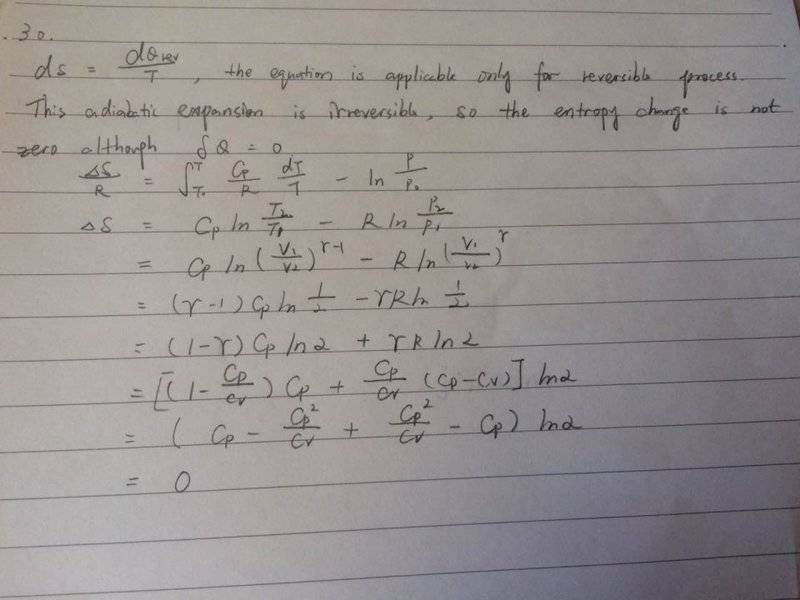# Irreversible adiabatic process - is the entropy change zero?

## Homework Statement

A well insulated container consists of two equal volumes separated by a partition - one half an ideal gas while the other is a vacuum. The partition is removed, and the gas expands. What is the entropy change per mole?

## Homework Equations

dS = dQrev/T
S/R = Cp/R dT/T - ln(P/P0)

## The Attempt at a Solution

As the process is irreversible, the entropy change should be non-zero even though there is no change in heat. However, after I perform the calculation with the aforementioned equation, I got zero as my final answer.I'm extremely confused about my result because I can't find any mistake in my calculation, while I do believe that the change should be non-zero.
Any help would be highly appreciated!

Chestermiller
Mentor

## Homework Statement

A well insulated container consists of two equal volumes separated by a partition - one half an ideal gas while the other is a vacuum. The partition is removed, and the gas expands. What is the entropy change per mole?

## Homework Equations

dS = dQrev/T
S/R = Cp/R dT/T - ln(P/P0)

## The Attempt at a Solution

As the process is irreversible, the entropy change should be non-zero even though there is no change in heat. However, after I perform the calculation with the aforementioned equation, I got zero as my final answer.
View attachment 96098
I'm extremely confused about my result because I can't find any mistake in my calculation, while I do believe that the change should be non-zero.
Any help would be highly appreciated!
For the irreversible process you are analyzing, you should have found that the change in temperature is zero, and the final pressure is half the original pressure. When you calculate the entropy change between the initial and final states, you then follow the following procedure:

1. Completely forget about the actual irreversible path between the initial and final states.
2. Devise a reversible path between these same two ends states. This reversible path does not need to bear any resemblance whatsoever to the actual process path. So, in your problem, for example, it doesn't have to be adiabatic. Any reversible path will do, since they will all give exactly the same result for the entropy change. For your problem, a reversible isothermal expansion might be a good choice.
3. Calculate the integral of dQ/T for the reversible path that you have identified. This will be ##\Delta S##

This is what they mean when they say ##\Delta S## is equal to the integral of dQrev/T.

Chet

•Ortanul